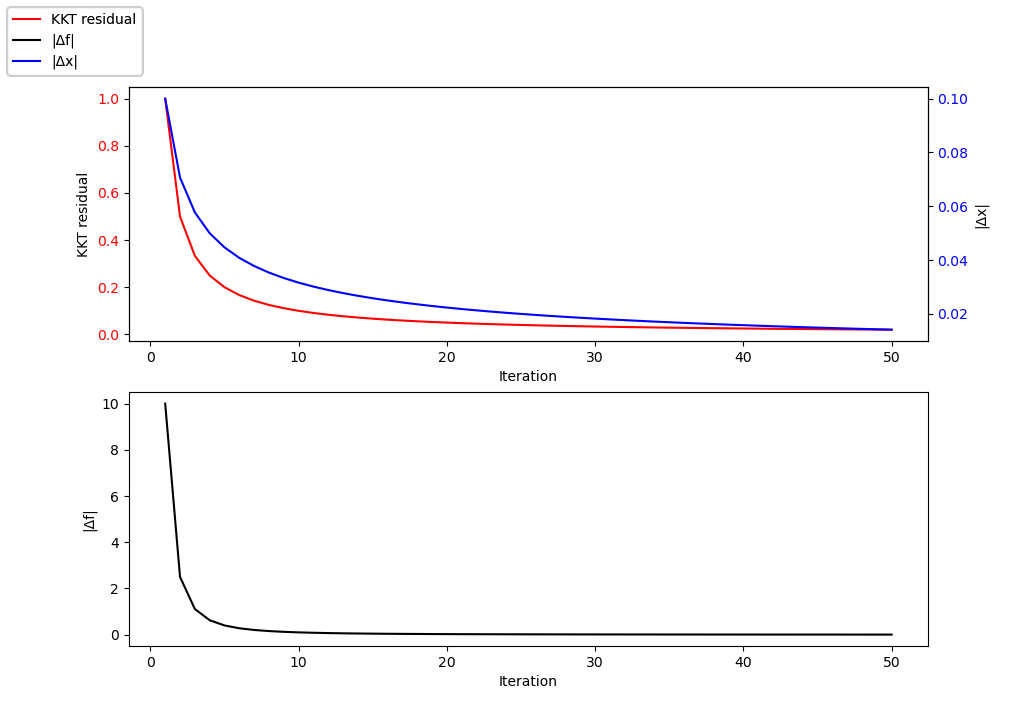## ConvergencePlots.jl

Visualizing convergence was never easier.
Author mohamed82008
Popularity
6 Stars
Updated Last
1 Year Ago
Started In
August 2020

# ConvergencePlots

This is a Julia package that makes it easy to do live tracking of the convergence of your algorithm. All the plotting is done using PyPlot.jl.

## Installation

To install `ConvergencePlots.jl`, run the following Julia code.

```using Pkg

## Usage

First create an empty plot:

`plot = ConvergencePlot()`

This will create an empty convergence plot that plots up to 100000 history points. Older points are overwritten. To specify how many history points to plot, use the constructor:

`plot = ConvergencePlot(n)`

where `n` is the number of points.

The keyword arguments you can pass to the `ConvergencePlot` constructor are:

• `names`: a `Vector{String}` that has all the names of the convergence metrics to be plotted. The default value of `names` is `["Residual"]`.
• `options`: a dictionary mapping each name in `names` to a `NamedTuple`. Each named tuple has the plotting options to pass to `PyPlot`, e.g. `(label = "KKT residual", ls = "--", marker = "+")`. If `label` is not passed, it defaults to the corresponding name in `names`. You can also pass a single `NamedTuple` of options without the `label` option, and it will be used for all the names.
• `show`: if `true` the empty figure will be displayed. This is `false` by default.

After creating an empty plot, you can add points to it as follows:

`addpoint!(plot, Dict("Residual" => 1.0))`

where the second argument can contain one value for each name in `names`. If only a single name exists, you can also use:

`addpoint!(plot, 1.0)`

Adding a point will display the plot by default. To stop the plot from displaying, set the `show` keyword argument to `false`.

To close the plot, call:

`closeplot!(plot)`

## Example

```using ConvergencePlots

plot = ConvergencePlot(
names = ["KKT residual", "|Δx|", "|Δf|"],
options = Dict(
"KKT residual" => (color = "red",),
"|Δx|" => (color = "blue",),
"|Δf|" => (color = "black",),
),
)
kkt = 1 ./ (1:50)
Δx = 0.1 .* sqrt.(kkt)
Δf = 10 .* kkt .^ 2
for i in 1:50
sleep(1e-4)
plot,
Dict(
"KKT residual" => kkt[i],
"|Δx|" => Δx[i],
"|Δf|" => Δf[i],
),
)
end```### Used By Packages

No packages found.